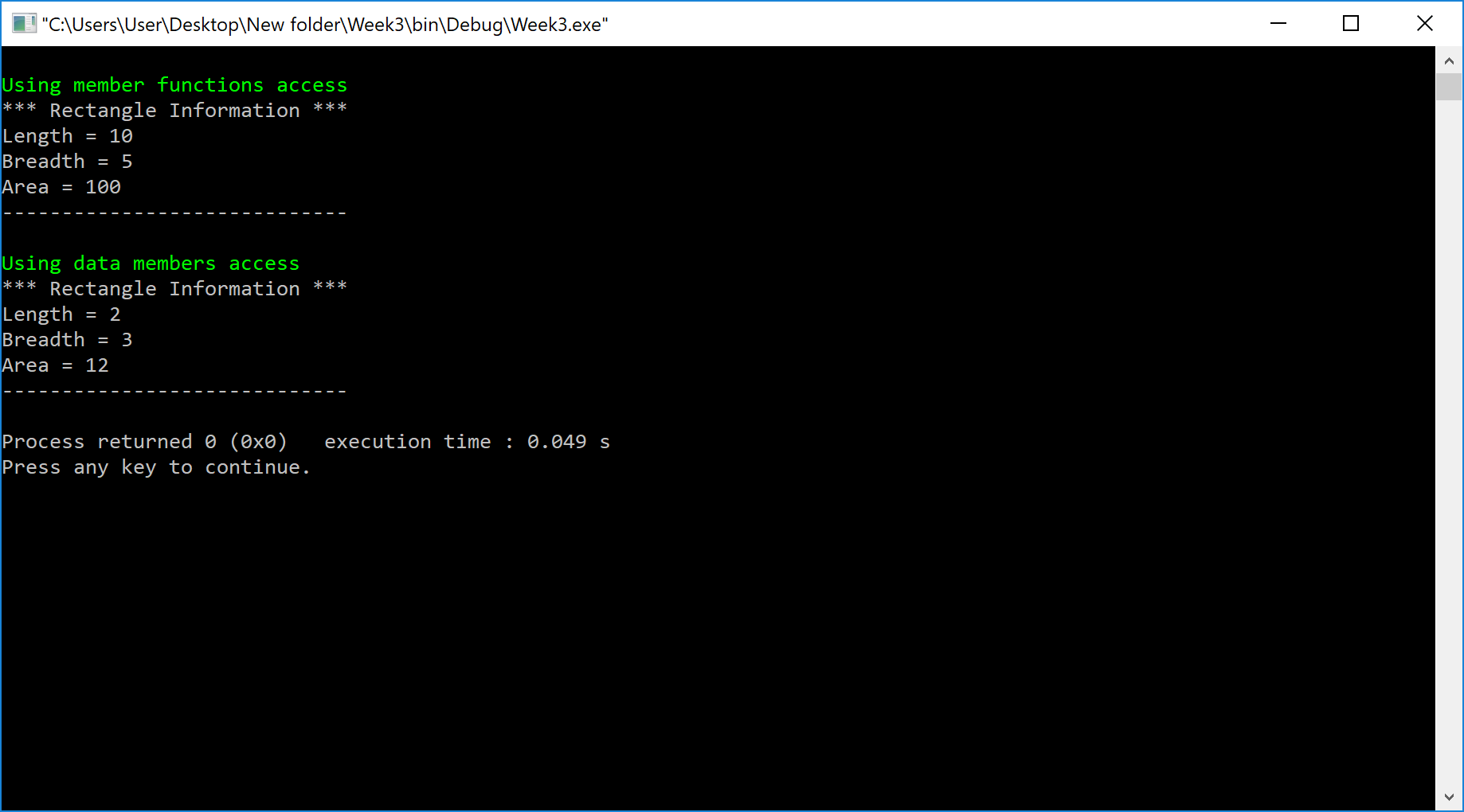The perfect place for easy learning...

×

# Aim

Write a C++ program to declare a class. Declare pointer to class. Initialize and display the contents of the class member.

# Procedure

• Step 1 - Include the required header files (iostream.h, conio.h, and windows.h for colors).
• Step 2 - Create a class (Rectangle) with the following members as public members.
• length and breadth as data members.
• initialize(), getArea() and display() as member functions.
• Step 3 - Create a main() method.
• Step 4 - Create a variable (rect) and a pointer variable (class_ptr) of the above class inside the main() method.
• Step 5 - Assign the address of class object (rect) to class pointer object (class_ptr).
• Step 6 - Then, call the member functions initialize() and display() using class pointer object (class_ptr) to illustrate member functions access using class pointer.
• Step 7 - Assign values to data members of the class using class pointer object to illustrate data members access using class pointer.
• Step 8 - Finally, call the member functions initialize() and display() using class pointer object (class_ptr).

# Implementation

C++ Program
``````#include <windows.h>
#include <iostream>

using namespace std;

class RectangleTest{

public:

public:
void initialize(int len, int bre){
length = len;
}
int getArea(){
}
void display(){
int area = getArea();
cout<<"\n*** Rectangle Information ***\n";
cout<<"Length = "<<length;
cout<<"\nArea = "<<area;
cout<<"\n-----------------------------\n";
}

};

int main()
{
RectangleTest rect, *class_ptr;
HANDLE color=GetStdHandle(STD_OUTPUT_HANDLE);
class_ptr = &rect;

//Accessing member functions using class pointer
SetConsoleTextAttribute(color, 10 );    //Setting color Green
cout<<"\nUsing member functions access";
SetConsoleTextAttribute(color, 7 );     //Setting color White
class_ptr->initialize(10,5);
class_ptr->display();

//Accessing data members using class pointer
SetConsoleTextAttribute(color, 10 );    //Setting color Green
cout<<"\nUsing data members access";
SetConsoleTextAttribute(color, 7 );     //Setting color White
class_ptr->length = 2;
class_ptr->display();

return 0;
}
```
```

# Result